# RD Sharma Solutions Chapter 15 Areas Related to Circles Exercise 15.1 Class 10 Maths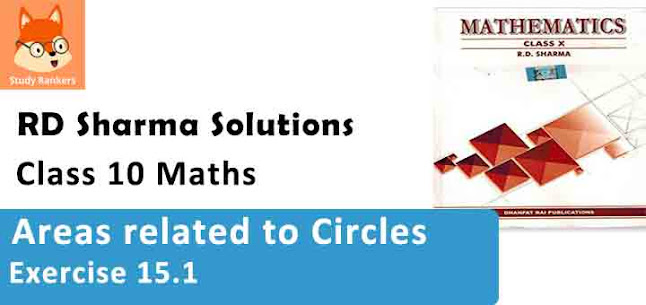Chapter Name RD Sharma Chapter 15 Areas Related to Circles Book Name RD Sharma Mathematics for Class 10 Other Exercises Exercise 15.1Exercise 15.2Exercise 15.3Exercise 15.4VSAQs and MCQs Related Study NCERT Solutions for Class 10 Maths

### Exercise 15.1 Solutions

1. Find the circumference and area of a circle of radius 4.2 cm.

Solution

Radius of a circle = 4.2 cm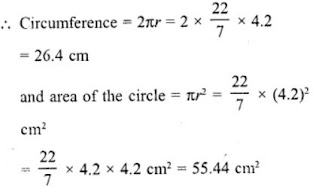2. Find the circumference of a circle whose area is 301.84 cm2.

Solution

Area of a circle = 301.84 cm2
Let r be the radius, then πr2 = 301.84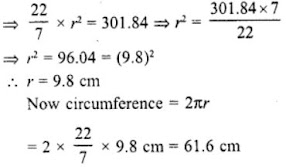3. Find the area of a circle whose circum­ference is 44 cm.

Solution

Circumference of a circle = 44 cm
then 2πr = circumference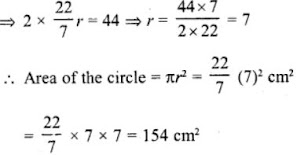4. The circumference of a circle exceeds the diameter by 16.8 cm. Find the circum­ference of the circle.

Solution

Let r be the radius of the circle
∴ Circumference = 2r + 16.8 cm
⇒ 2πr = 2r + 16.8
⇒ 2πr – 2r = 16.8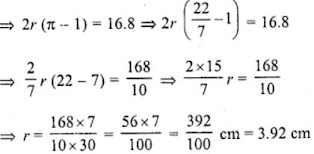Circumference = 2r + 16.8
= 2×3.92 + 16.8 cm
= 7.84 + 16.8 cm
= 24.64 cm

5. A horse is tied to a pole with 28 m long string. Find the area where the horse can graze. (Take π = 22/7)

Solution

Radius of the circle (r) = Length of the rope = 28 m.
Area of the place where the horse can graze be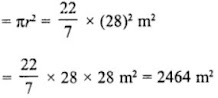6. A steel wire when bent in the form of a square encloses an area of 121 cm2. If the same wire is bent in the form of a circle, find the area of the circle.

Solution

Area of square formed by a wire =121 cm2
∴ Side of square (a) = √Area = √121 = 11 cm
Perimeter of the square = 4×side = 4×11 = 44 cm
∴ Circumference of the circle formed by the wire = 44cm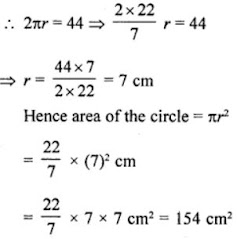7. The circumference of two circles are in the ratio 2 : 3. Find the ratio of their areas.

Solution

Let R and r be the radii of two circles and their ratio between them circumference = 2 : 3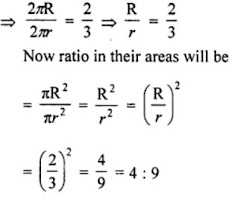8. The sum of radii of two circles is 140 cm and the difference of their circum­ferences is 88 cm. Find the diameters of the circles.

Solution

Let R and r be the radii of two circles Then R + r = 140 cm …(i)
and difference of their circumferences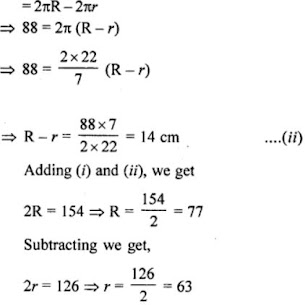∴ First diameter = 2R = 2×77 = 154 cm
∴ Second diameter = 2r = 2×63 = 126 cm

9. Find the radius of a circle whose circumference is equal to the sum of the circumferences of two circles of radii 15 cm and 18 cm.

Solution

Let the radius of a circle be r.
Circumference of a circle = 2πr
Let the radii of two circles are r1 and r2 whose
values are 15 cm and 18 cm respectively.
i.e., r1 = 15 cm and r2 = 18 cm
Now, by given condition,
Circumference of circle = Circumference of first circle + Circumference of second circle
⇒ 2πr = 2π r1 + 2πr2
⇒ r = r1 + r2
⇒ r = 15 + 18
∴ r = 33 cm
Hence, required radius of a circle is 33 cm.

10. The radii of two circles are 8 cm and 6 cm respectively. Find the radius of the circle having its area equal to the sum of the areas of the two circles.

Solution

Radius of first circle (r1) = 8 cm
and radius of second circle (r2) = 6 cm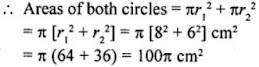Let R be the circles whose area is the sum of given two circles.
Then, area = πR2
πR2 = 100π
⇒ R2 = 100 = 102
⇒ R = 10 cm
∴ Radius of the required circle = 10 cm

11. The radii of two circles are 19 cm and 9 cm respectively. Find the radius and area of the circle which has its circumference equal to the sum of the circumferences of the two circles.

Solution

Radius of the first circle (r1) = 19 cm
and radius of the second circle (r2) = 9 cm S
um of their circumferences = 2πr1 + 2πr2
= 2π (r+ r2) = 2π (19 + 9) cm
= 2π × 28 = 56π cm
Let R be the radius of the circle whose circumference is the sum of the circum­ferences of given two circles, then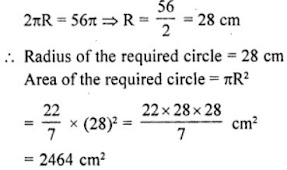12. The area of a circular playground is 22176 m2. Find the cost of fencing this ground at the rate of ₹50 per metre.

Solution

Given, area of a circular playground = 22176 m2
r2 = 22176 [area of circle r2]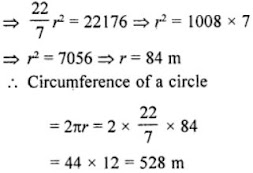∴ Cost of fencing this ground = 528 50 = 26400

13. The side of a square is 10 cm. Find the area of circumscribed and inscribed circles.

Solution

ABCD is a square whose each side is 10 cm
∴ AB = BC = CD = DA = 10 cm
AC and BD are its diagonals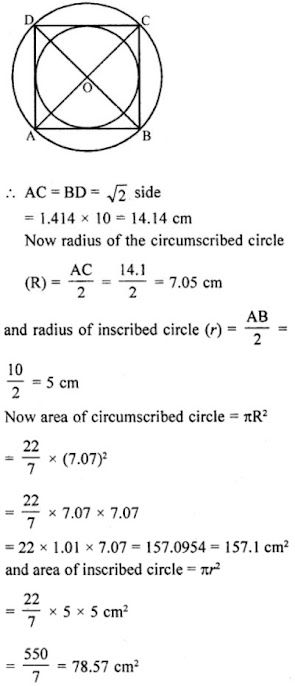14. If a square is inscribed in a circle, find the ratio of the areas of the circle and the square.

Solution

Let r be the radius of the circle a be the side of the square